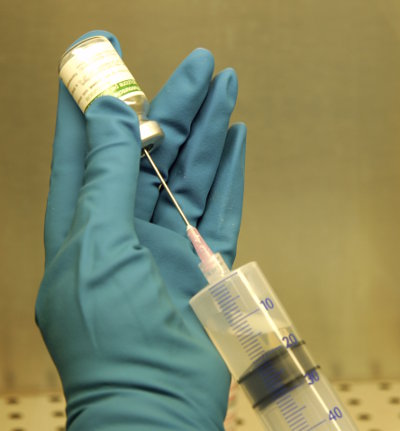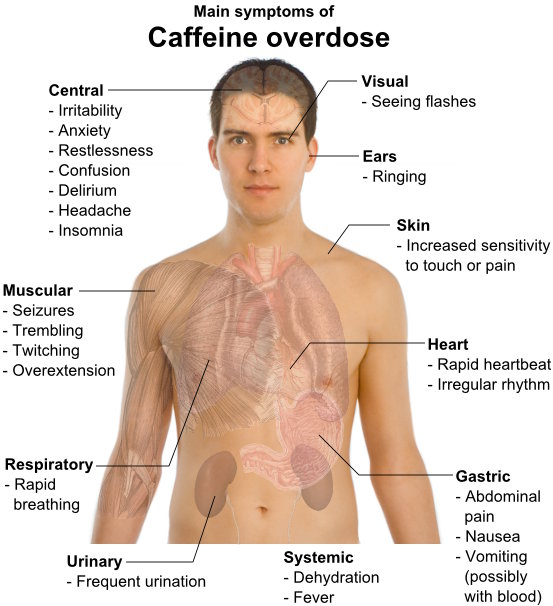# Direct Proportion

Direct proportion is when two quantities change in the same way.

If you can multiply the first quantity by the same number (called a constant) to get the second quantity, they are in direct proportion.

For example, if 1 ice-cream costs \$2, then 2 ice-creams will cost \$4, 3 ice-creams cost \$6, 4 ice-creams cost \$8 and so on. You can multiply the number of ice-creams by 2 to get the cost of the ice-creams, so the number of ice-creams and the cost of ice-creams must be in direct proportion.

Other examples of direct proportion are:

• the cost of apples at \$5 per kilogram
• total wages earned at \$20 per hour
• amount of flour needed to make muffins (at 3 cups of flour for every 10 muffins)
• distance travelled and fuel used (assuming the car speed is the same).

## Example One - Travel

A car travels 100 km in 1 hour. How far will it travel in 3 hours?

The multiplying constant is 100.
3 × 100 = 300 km

## Example Two - Concrete

Four bags of cement are needed to make 1 cubic metre of concrete. How many bags are needed to make 5 cubic metres of concrete?

The multiplying constant is 4.
5 × 4 = 20 bags

## Example Three - MedicationA sick child whose mass is 14 kg should receive medication dose that is in proportion to the dose of a 70 kg adult. If the adult dose is 10ml, how much should the child receive?

The multiplying constant is 70 ÷ 14 = 5.
10 ÷ 5 = 2 ml.

## Questions

Q1. In adult cod fish who are constantly consuming seawater as they swim, their kidneys make urine at a constant rate of 50 millilitres every hour. How much urine does a cod fish make and excrete into the ocean in 5 hours?

Q2. Malik, a talented muffin maker, uses 2 cups of blueberries in every batch of muffins. How many cups of blueberries does he use when he bakes 6 batches?

Q3. My computer can download 100 MP3 tracks in 2 hours, how many can it download in 8 hours? (Hint: Find the multiplying constant, that is, the number of MP3s downloaded in 1 hour.)

Q4. A sick child whose mass is 28 kg receives a proportional dose to a 70 kg adult's dose of 100ml. What is the child's dosage?

Q5. In 1998, Australian Olympic gold medallist won the 200 metres freestyle in 1 minute 46.7 seconds and then the 400 metres freestyle in 3 minutes 44.35 seconds. Are these in direct proportion? (Hint: Work out the time for swimming 100 metres in each race.)